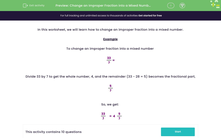# Change an Improper Fraction into a Mixed Number

In this worksheet, students will change an improper fraction into a mixed number.Key stage:  KS 3

Curriculum topic:   Number

Curriculum subtopic:   Use Four Operations for All Numbers

Difficulty level:#### Worksheet Overview

In this activity, we will learn how to change an improper fraction into a mixed number.

To change an improper fraction into a mixed number, we need to divide the numerator by the denominator.

The answer gives us the whole number and the remainder is written as the new numerator

Here's an example:

 33 7

First of all, we need to divide 33 by 7. This gives us 4, remainder  5. (33 - 28 = 5)

The 4 is the whole number and we write the remainder as the new numerator.

So, we get:

 33 7
= 4
 5 7

Let's try another one.

What is 29/5 as a mixed number?

First of all, we divide the numerator by the denominator: 29 ÷ 5 = 5 remainder 4

The 5 is the whole number and the remainder becomes the new numerator: 5 4/5

Are you ready to have a go at some questions now?### What is EdPlace?

We're your National Curriculum aligned online education content provider helping each child succeed in English, maths and science from year 1 to GCSE. With an EdPlace account you’ll be able to track and measure progress, helping each child achieve their best. We build confidence and attainment by personalising each child’s learning at a level that suits them.

Get started